• 2019-11-30 11:51:08

# 理论部分：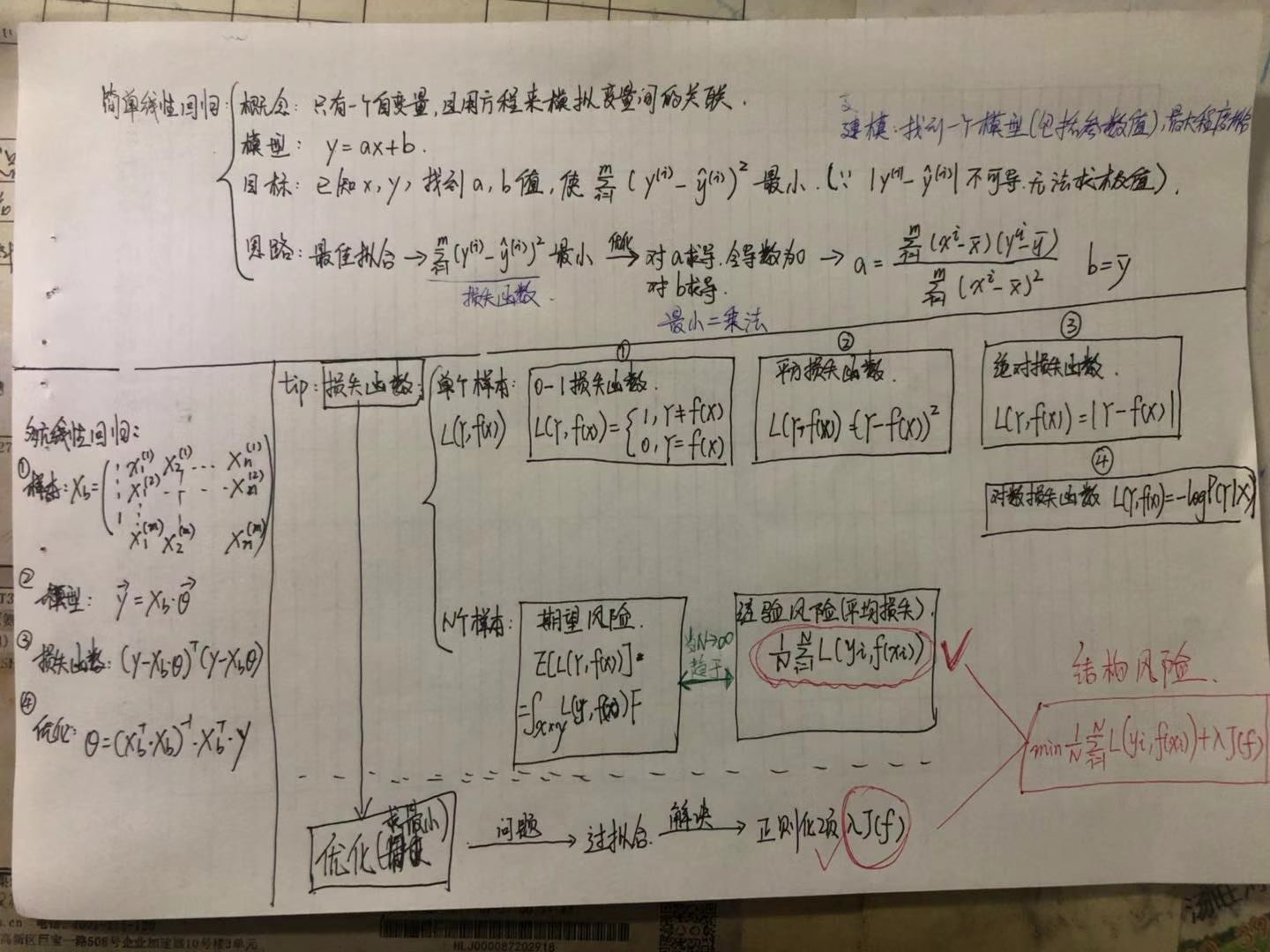# 代码部分：

from myAlgorithm.SimpleLinearRegression import SimpleLinearRegression

x = np.array([1.,2.,3.,4.,5.])
y = np.array([1.,3.,2.,3.,5,])
x_predict = np.array()
reg = SimpleLinearRegression()
reg.fit(x,y)

import numpy as np
import matplotlib.pyplot as plt
from sklearn import datasets

X = boston.data
y = boston.target

X = X[y<50.0]
y = y[y<50.0]

X.shape
输出：(490, 13)

y.shape
输出：(490, )

from myAlgorithm.model_selection import train_test_split
from myAlgorithm.LinearRegression import LinearRegression

X_train, X_test, y_train, y_test = train_test_split(X, y, seed = 666)

reg = LinearRegression()
reg.fit_normal(X_train, y_train)

reg.coef_
输出：
array([-1.18919477e-01,  3.63991462e-02, -3.56494193e-02,  5.66737830e-02,
-1.16195486e+01,  3.42022185e+00, -2.31470282e-02, -1.19509560e+00,
2.59339091e-01, -1.40112724e-02, -8.36521175e-01,  7.92283639e-03,
-3.81966137e-01])

reg.interception_
输出：
34.16143549622471

reg.score(X_test, y_test)
输出：
0.81298026026584658

更多相关内容
• C++实现多元线性回归 可任意指定几元 根据输入的数据矩阵，和给定的回归元数，训练给出回归方程式
• 最小二乘法是先将方程自变量与因变量化为系数矩阵X，再求该矩阵的转置矩阵（X1），接着求矩阵X与他的转置矩阵的X1的乘积（X2），然后求X2的逆矩阵。最后整合为系数矩阵W，求解后分别对应截距b、a1、和a2。可见计算一...
• 一、多元线性回归函数；二、使用梯度下降法处理多元线性回归函数；三、特征缩放；四、学习率α；五、特征和多项式回归；六、正规方程

## 一、多元线性回归函数

上面解决了只有单一变量（面积）的房子价格预测问题，但是如果存在多个特征如：面积、卧室数量、楼层、修建年限等多个特征，如下：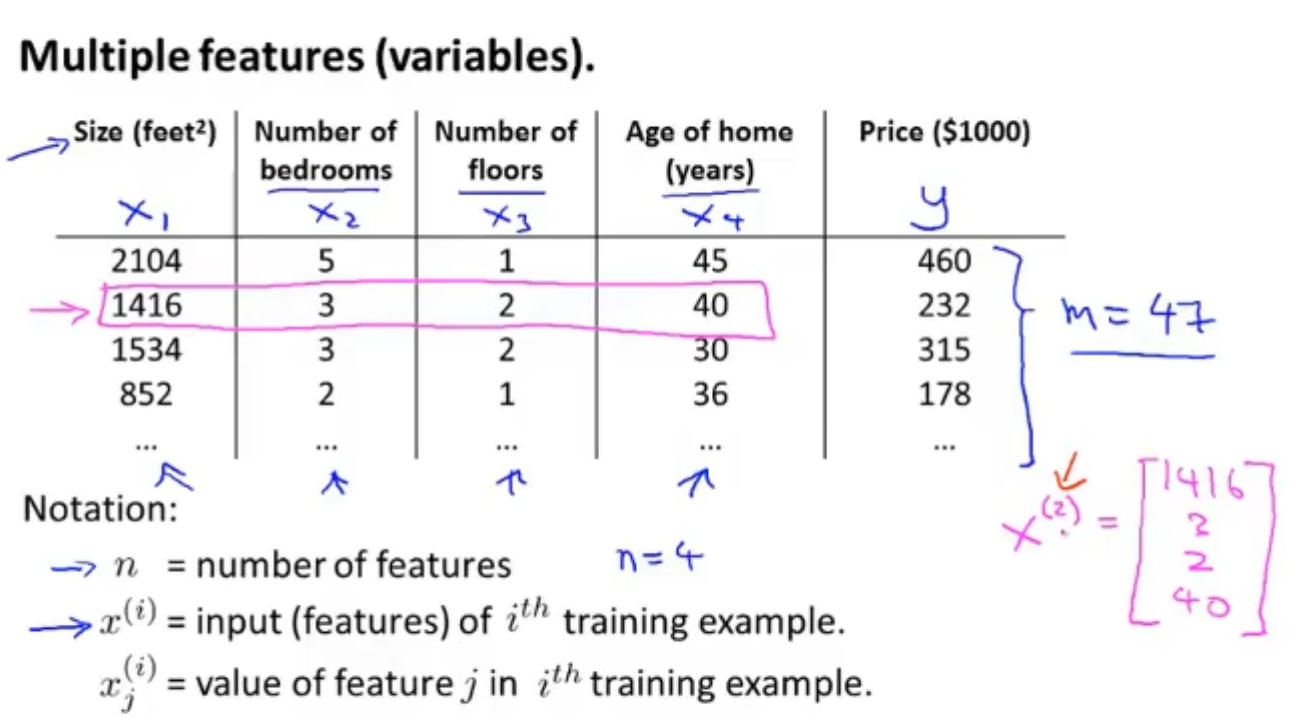我们用m表示样本数量，n表示特征数量，表示输入的第i个样本，表示输入的第i个样本的第j个特征。

只有一个变量时假设函数是：，现在我们有4个特征，所以假设函数变为：，我们引入=1，则方程可以变为：，写成矩阵形式为：

则

这就是多特征的线性回归函数，我们称为多元线性回归函数。

## 二、使用梯度下降法处理多元线性回归函数

我们可以使用梯度下降处理一元线性回归函数的方法处理多元线性回归函数，如下图，左边是一元，右边是多元，注意其中的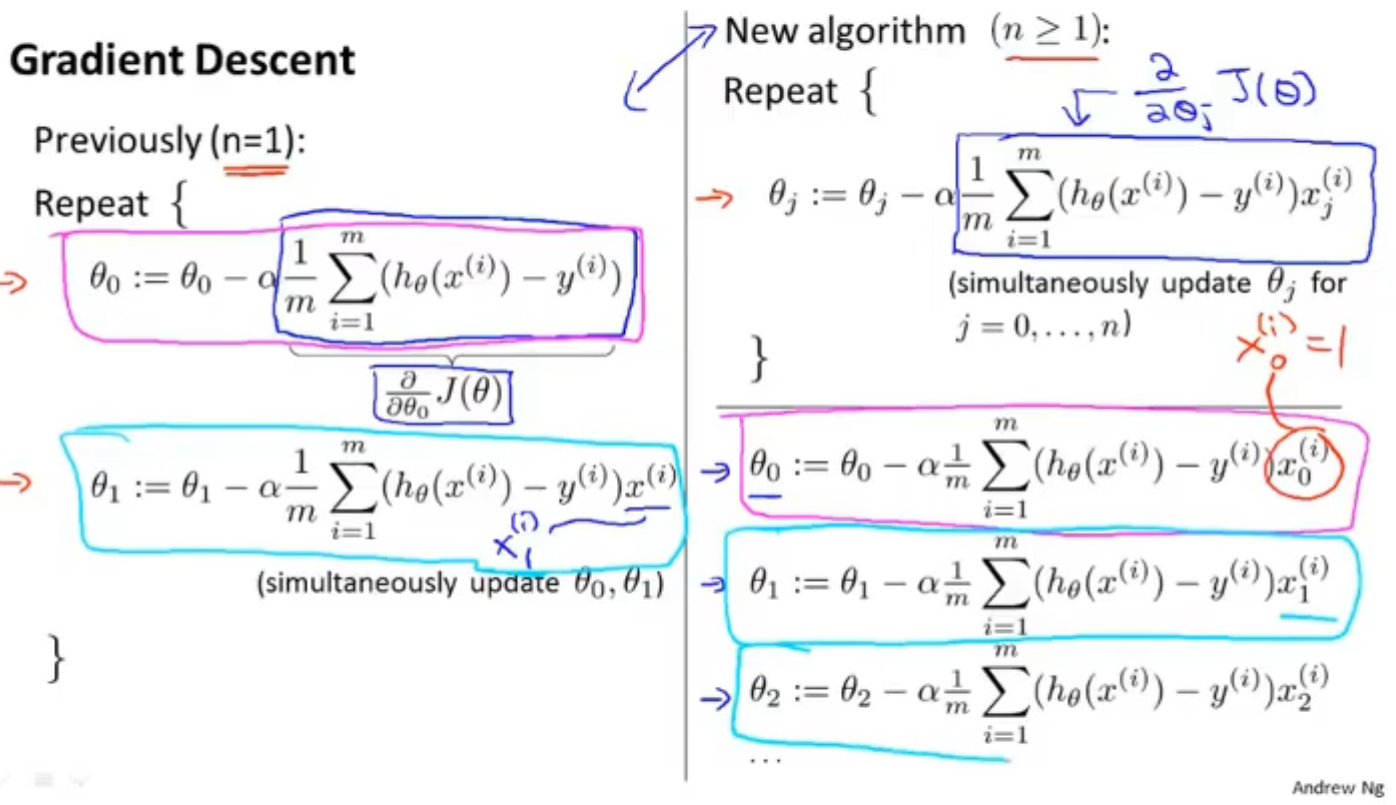## 三、特征缩放

在进行梯度下降算法时，如果存在多个特征差距很大，比如房屋的卧室数量范围是1-5，面积值范围是500-2000，那么在进行梯度下降时，由于同样的步长，面积产生的数值更大，对结果的影响更大会导致函数的下降过程来回摇摆，收敛速度变慢。我们采用缩放的方法，将卧室数量/5，面积/2000，得到一个0和1之间的数值，那么下降过程就会顺滑很多，也会更快。如下：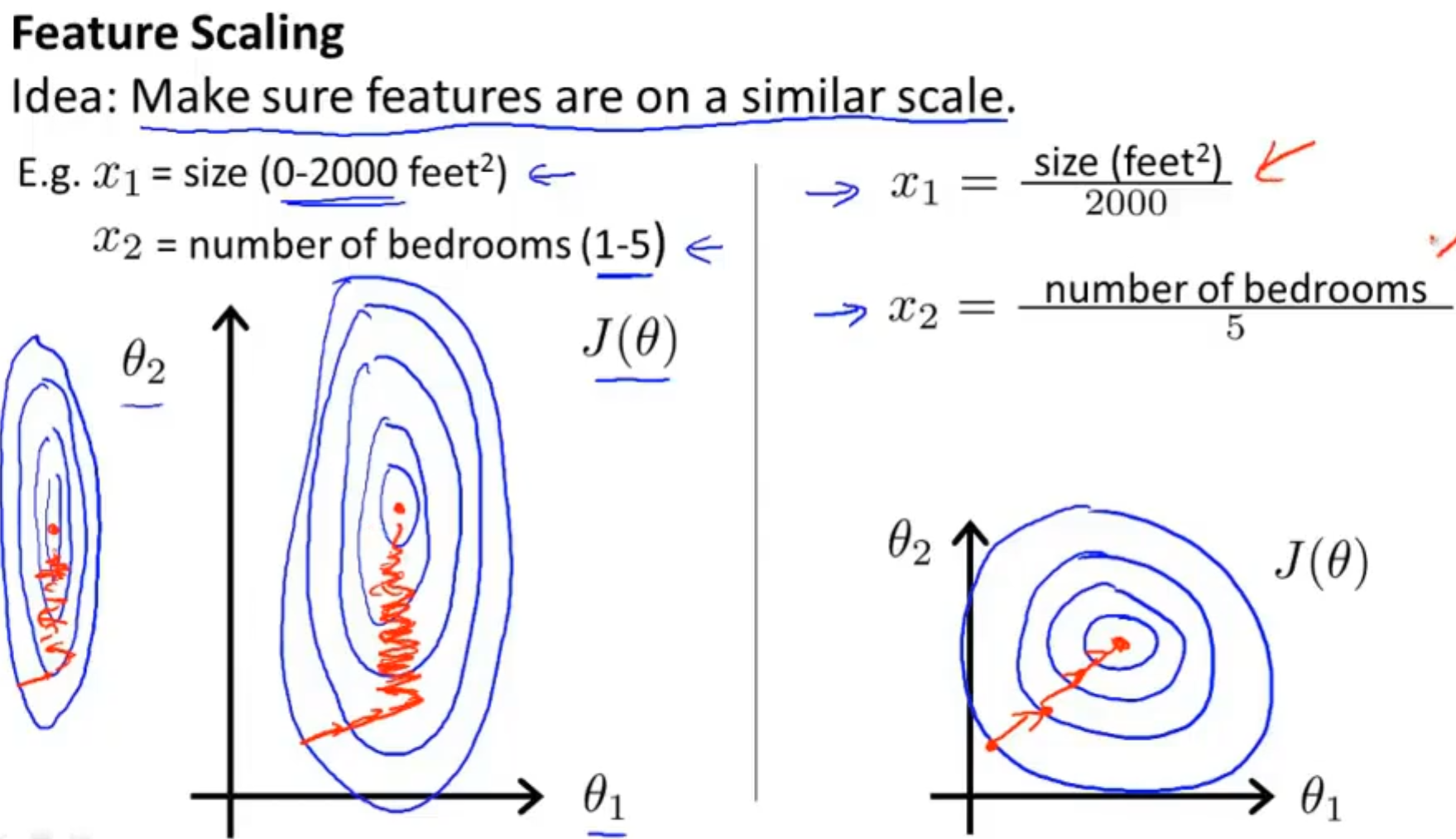但是，要注意，如果特征值的范围过小时也要适当放大。特征值不用都在同一区间，但彼此之间的差距不要太大。

均值归一化：（实际值-平均值）/最大值。经过均值归一化处理的数据能保证平均数为0的均匀分布。

## 四、学习率α

我们可以把梯度下降法的迭代次数作为横坐标，作为纵坐标，观察梯度下降算法是否收敛。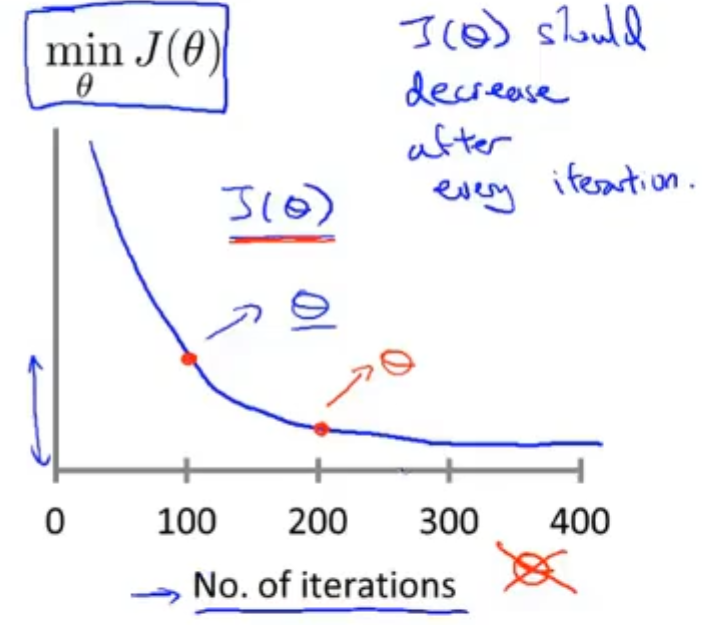在迭代一定的次数之后，函数下降的幅度很小，几乎为一条直线。此时就可以看做函数已经收敛。

另外，也可以进行一种收敛的测试，设定一个阈值，如果代价函数一步迭代后的下降小于这个阈值，那么就可以认为函数收敛了。但是通常一个合适的阈值是难以确定的。曲线图会比较直观。

学习率α过大，会出现下面这样的迭代次数-代价函数图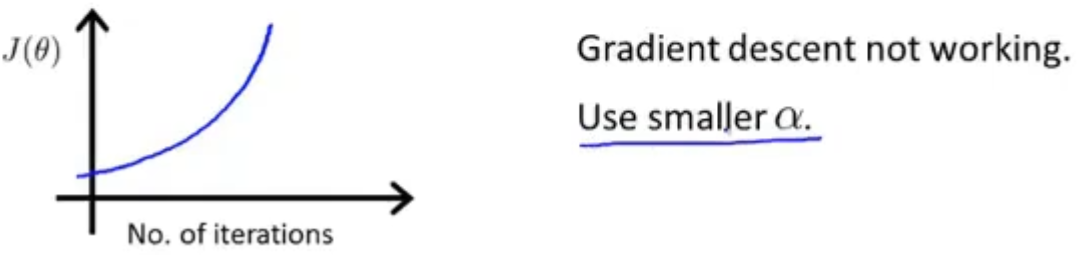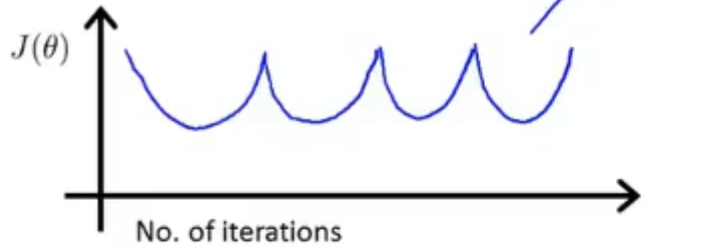总结：学习率α过小，会出现收敛速度慢的问题；过大，会出现代价函数不会每次迭代都下降甚至不收敛

## 五、特征和多项式回归

### （一）如何选取特征

假设我们想卖出的房子的图片长度为20米，宽度为10米。我们就有两个特征长和宽，但是我们使用线性回归算法的时候并不一定要直接使用这两个特征，我们可以自己创造新的特征，我们实际观察到房子的价格其实与房子的面积关系最大，我们可以使area=长度*宽度，这就是房子的面积，两个特征变成了一个新特征。

### （二）选取不同的函数作为代价函数

比如有下面这样一个模型，直线不能很好的拟合。我们可以用二次模型去拟合，但是二次函数会降下来，不合理。我们不觉得随着土地面积的增加，房子价格会降下来。我们使用一个三次函数去预测，如图中绿色曲线。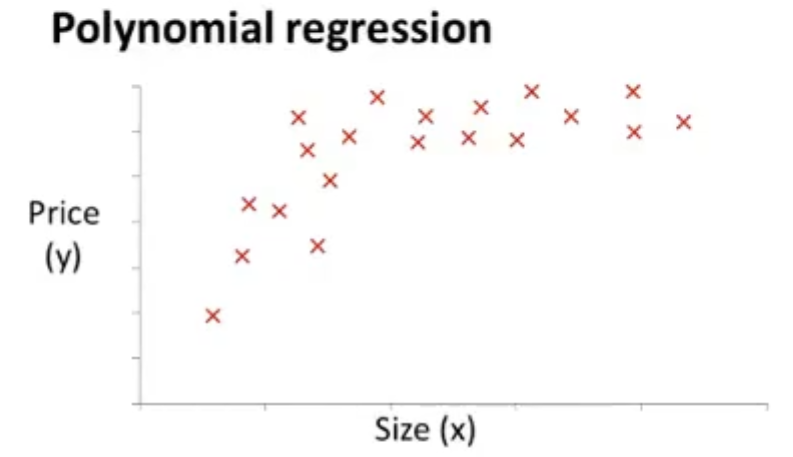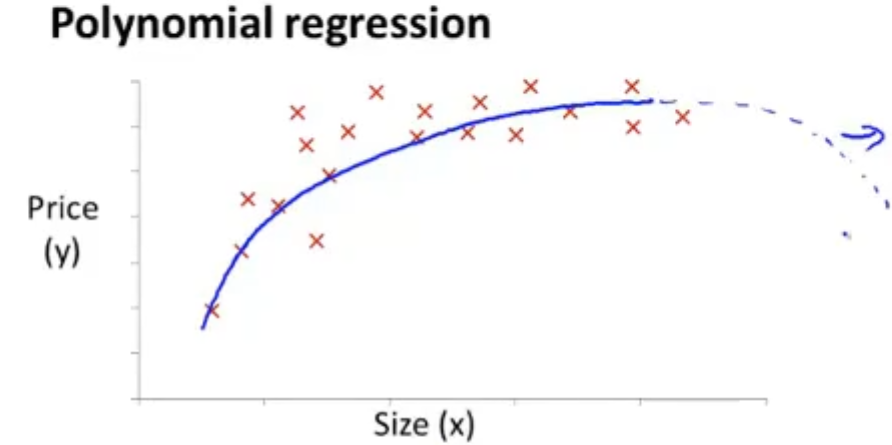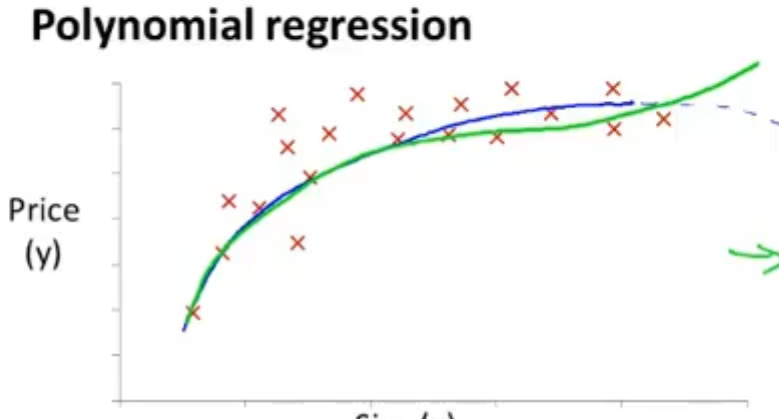那么假设函数就如下所示：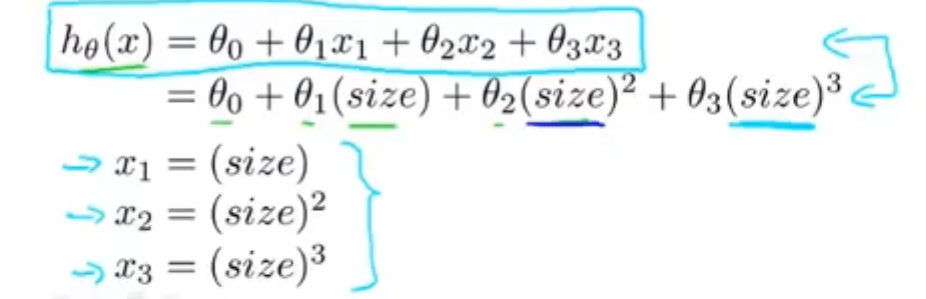## 六、正规方程

### （一）正规方程求解线性回归函数

设有一个数据集如下所示，x1,x2,x3是特征，y为标签：

x1x2x3y
x11x12x13y1
x21x22x23y2

设假设函数为，其中，即在数据集上加上一列全为1的x1，如下：

则用矩阵的方式表示可得：等价于，其中：

，等价于，现在我们要求解向量。因为x矩阵不是方阵，只有方阵才能求逆矩阵，所以我们需要把x转换为方阵，两边同时左乘x矩阵的转置，得：，其中肯定是一个方阵，把它看做一个整体，两边同时右乘它的逆矩阵得：，此时就求出了向量。

使用特征方程的话就不需要特征缩放了

### （二）特征方程的优缺点

假设有m个样本，n个特征。

梯度下降 特征方程 优点 不需要多次迭代 不需要选择学习速率α 缺点 当n特别大时需要计算，这是一个n*n的矩阵，运算速度慢，时间复杂度大约是O() 优点 当n特别大时能很好的运行 其中n的大小很难给出准确数字，但现在计算机的速度n在万级以下没有问题 缺点 需要选择学习速率α 需要多次迭代

对于一些复杂的方法，如一些分类算法，是无法使用特征方程求解的，我们只能使用梯度下降法。

### （三）正规方程以及不可逆性

对于，如果不可逆，如何求解？我们把不可逆的矩阵称为退化矩阵或奇异矩阵，在实际情况中不可逆的情况很少见。矩阵求逆有两种，分别为：伪逆(pseudo-inverse)和逆(inverse)，数学上证明，即使矩阵是不可逆的，利用伪逆也可以求出结果。

（1）一般出现不可逆的情况

①冗余的特征

如 x1=平方英尺，x2=平方米，那么两个特征之间有换算关系即 x1=3.28.3.28*x2，两列成倍数则矩阵不可逆

②特征数n大于样本数m

删除一些特征或使用正则化方法可以解决这个问题

展开全文机器学习 sklearn 算法
• 下面是多元线性回归用Python实现的代码： import numpy as np def linearRegression(data_X,data_Y,learningRate,loopNum): W = np.zeros(shape=[1, data_X.shape]) # W的shape取决于特征个数，而x的行是样本...
• 包括基于矩阵运算的多元线性回归、基于多元计算程序包以及计算共线性容忍度
• Mathematica在多元线性回归分析中的应用，黄志鹏，李思泽，从多元线性回归分析的原理出发，利用最小二乘法准则，将回归分析归结为用Seidel迭代法求矩阵方程组解，确定待定系数，利用mathematica�
• 多元线性回归表达式 其中， X=[1,x1,⋯ ,xn] X=[1,x_1,\cdots,x_n] X=[1,x1​,⋯,xn​] W=[w0,w1,⋯ ,wn] W=[w_0,w_1,\cdots,w_n] W=[w0​,w1​,⋯,wn​] 损失函数 其中X,YX,YX,Y是矩阵。 梯度下降 令H=Y−XWTH=...

# 多元线性回归表达式其中，
X = [ 1 , x 1 , ⋯   , x n ] X=[1,x_1,\cdots,x_n]

W = [ w 0 , w 1 , ⋯   , w n ] W=[w_0,w_1,\cdots,w_n]

# 损失函数其中 X , Y X,Y 是矩阵。

# 梯度下降

H = Y − X W T H=Y-XW^T
L = H T H L=H^TH这里涉及到了矩阵求导的知识，具体请查看
矩阵求导

# 代码实现

import numpy as np
import matplotlib.animation as animation
import matplotlib.pyplot as plt
# 以下两行代码解决jupyter notebook显示图片模糊问题
%matplotlib inline
%config InlineBackend.figure_format = 'svg'
# 支持中文
plt.rcParams['font.sans-serif'] = ['SimHei']  # 用来正常显示中文标签
plt.rcParams['axes.unicode_minus'] = False  # 用来正常显示负号
##  测试数据
x1 = np.arange(0,10,0.2)
x2 = np.arange(-10,0,0.2)
y = 10*x1+3*x2+5 + np.random.random((len(x1)))*20

def f(X,W):
return X.dot(W.T)
## 初始化参数
W =np.mat(np.random.random()*2-1)
X=np.mat(np.column_stack((np.ones(len(x1)),x1,x2))) #按列组合
Y = np.mat(y).T

## 梯度下降
lr = 0.00005
for i in range(60):
# 更新梯度
w = -2*(Y-X.dot(W.T)).T.dot(X)
W = W-lr*w
# 绘图
Y_Test = f(X,W)
x = np.arange(len(Y))+1
plt.scatter(x,list(Y),s=10)
plt.plot(x,Y,'b')
plt.plot(x,Y_Test,'r')展开全文python 机器学习
• 控制台应用程序，C++实现多元线性回归，可指定任意几元，根据给定的数据矩阵，训练给出回归方程式
• 一元线性回归的公式为 y = a*x + b, 多元线性回归的公式与一元线性回归的公式类似，不过是矩阵形式，可以表示为Y =　AX + b，其中，Y是样本输出的合集，X是样本输入的合集。多元线性回归最终求解的内容也就是 A 和...

多元线性回归法也是深度学习的内容之一，用java实现一下多元线性回归。

一元线性回归的公式为 y =  a*x + b, 多元线性回归的公式与一元线性回归的公式类似，不过是矩阵的形式，可以表示为Y =　AX + b，其中，Y是样本输出的合集，X是样本输入的合集。

多元线性回归最终求解的内容也就是 A 和 B， 这里省去求证步骤，直接列出求解 A 和 B 矩阵的公式，公式如下：这里的X是样本输入的合集，是二维矩阵，Y是样本输出的合集，是一维矩阵。

可以看到，这里要求解两次，先将 A 求出来，再将 b 求出来，这样会增加计算量，也增加了编程时的困难，实际上，如果我们将多元线性回归的方程展开来看：可以看到，如果我们将 b <=> a0 , 那么线性回归方程可以表示为：这样的话，矩阵的求解公式可以表示为：这里的 X 是一个新的矩阵，增加了“第零列”元素，该列元素全部设置为1。

现在可以进行编程，编程过程可以分为两步，第一步，给 X 矩阵增加“第零列”， 第二步， 求解 A 矩阵。

第一步的代码如下，这里我设置了一个多元线性回归类，将传进来的 X 和 Y，赋值给类中的 X 和 Y：

	/**如果采用无参构造，则需要通过set输入相应的矩阵
* @param X x的矩阵
* @param Y y的矩阵
*/
public void set(double X[][], double Y[]){
this.X = new double[X.length][X.length + 1];
for(int i = 0; i < X.length; i++){
this.X[i] = 1;
for(int j = 0; j < X.length; j++){
this.X[i][j + 1] = X[i][j];
}
}
this.Y = Y;
}
完成后，求解 A 矩阵， 因为公式是矩阵形式，所以直接采用设计好的矩阵计算类进行求解，这里用到的主要是矩阵的转置，求逆以及矩阵的乘法，矩阵求逆和乘法在之前的两篇博客中列出，矩阵求逆： 点击打开链接，矩阵的乘法： 点击打开链接，因为这次计算只需要用到二维矩阵的转置，所以这里只列出二维矩阵的转置：
	/**求转置矩阵
* @param A 要求解的矩阵
* @return 转置矩阵
*/
public double[][] matrix_transpoistion(double A[][]){
int row = A.length;
int col = A.length;
double A_T[][] = new double[col][row];
for(int i = 0; i < row; i++){
for(int j = 0; j < col; j++){
A_T[j][i] = A[i][j];
}
}
return A_T;
}

A 矩阵的求解公式列在这里：也就是说，整个求解过程分为五步，(1)求转置，(2)转置与原矩阵的乘积，(3)乘积的逆，（4）结果乘矩阵的转置，(5)结果乘输出矩阵，考虑到 X 的转置要用两次，可以预设一个 X_T 矩阵，保存 X 的转置，整个求解过程的实现代码如下：
	/**计算相应的系数
* @return 包含b的系数组
*/
public double[] cal_coefficient(){
int row = X.length;
int col = X.length;
//使用矩阵计算相应结果
Matrix_cal mc = new Matrix_cal();
//求X的转置
double X_T[][] = mc.matrix_transpoistion(X);
//求XT*X;
mc.set(X_T);
double temp[][] = mc.matrix_multriple(X);
//求逆矩阵
if(temp.length > 1){
//如果X_T*X的矩阵行列数不为1
mc.set(temp);
temp = mc.inverse_Matrix();
}
else{
//如果矩阵行列式为1，则temp不变
temp = 1/temp;
}
//求temp*X_T;
mc.set(temp);
temp = mc.matrix_multriple(X_T);
//mc.show(temp);
//求temp*Y
mc.set(temp);
B = mc.matrix_multriple(Y);
return B;
}
}
这里的 Matrix_cal，是我之前所写的一个基本的矩阵运算类，最后返回的结果是一个一维矩阵，也就是需要计算的 A。

展开全文java Matrix
• 多元线性回归模型： y = b1x1 + b2x2 + b3x3 +…… +bnxn; 我们根据一组数据： 类似 arr_x = [[1, 2, 3, 4, 5], [6, 7, 8, 9, 10], [11, 12, 13, 14, 15]]; arr_y = [5, 10, 15]; 我们最后要求出的是一个数组，包含...
• ## 多元线性回归推导过程

万次阅读 多人点赞 2018-08-06 20:26:23
常用算法一 多元线性回归详解1 此次我们来学习人工智能的第一个算法:多元线性回归.文章会包含必要的数学知识回顾,大部分比较简单,数学功底好的朋友只需要浏览标题,简单了解需要哪些数学知识即可. 本章主要包括...人工智能 最小二乘法
• ## 多元线性回归算法

千次阅读 2021-10-25 18:39:30
文章目录一、概念二、EXCEL的多元线性回归三、代码实现多元线性回归1.sklearn包实现2.线性回归模型的统计学库实现四、总结参考链接 一、概念 在回归分析中，如果有两个或两个以上的自变量，就称为多元回归。事实上...回归
• 线性回归是回归分析的一种，评估的自变量X与因变量Y之间是一种线性关系，当只有一个自变量时，成为简单线性回归，当具有多个变量时，称为多元线性回归。 线性关系的理解： >画出来的图像是直的（简单线性回归...算法 回归
• 两自变量的值输入X1、X2矩阵，因变量的值输入Y1矩阵。循环计算多元线性回归
• 多元线性回归 一、多元线性回归理解 通过一元线性回归的实现和推理，我清楚了一元线性回归的用法 然后来到多元线性回归 线性，图像上任意一点的坐标,y值都是x值的a倍.我们把这种横纵坐标始终呈固定倍数的关系叫做...机器学习
• 有很多初学者不知道如何用R语言做回归，这里我讲解一下简单线性回归和多元线性回归。 当回归模型包含一个因变量和一个自变量时，我们称为简单线性回归。比如：身高和体重的关系。 当有不止一个预测变量时， 则...回归 机器学习
• 上一篇我们详细的讲解了一元一次线性回归算法，今天我们为大家讲解多元线性回归是怎么一回事。 何为多元？当我们的输入x只有一维属性时，我们称之为一元。就像我们判断人胖瘦，只需了解体重这一个属性，我们就可以...机器学习 算法 人工智能
• 文章目录1，概念2，用Excel进行多元线性回归1，删掉表里的不需要的项，即非数据项2，分析数据库3，代码方式实现多元线性回归1，导入包2，读取文件3，取出变量4，进行多元线性回归并得出结果5，结果6，检测异常7，...回归 算法
• 对t p>[t] F R^2 置信区间的值做出相应解释 因变量是新生儿体重birth weight 主题是产前护理及父母恶习对新生儿健康的影响 此外hypothesis假设应该...不过受制于原始数据，一般都不完美，回归的方程都这样鸟样，...
• 利用矩阵形式多元线性回归模型的进行估计、检验并分析了实现的具体步骤。结合实例建立了回归模型,利用该模型给出了回归系数的置信区间、因变量平均值的置信区间、因变量个别值的预测区间及多元线性估计回归模型...
• 一、EXCEL进行多元线性回归 1.首先需要下载一个数据分析的插件： 点击左上角文件->选项->加载项->分析工具库->转到-数据分析库->确定 下载好插件之后就可以看到这里多了一个数据分析 点击...回归 算法
• 0.多元线性回归多元线性回归是统计学中经常用到回归方法，一般需满足一下六个条件：随机误差项是一个期望值或平均值为0的随机变量；对于解释变量的所有观测值，随机误差项有相同的方差；随机误差项彼此不相关；解释...
• 《利用MATLAB进行多元线性回归》由会员分享，可在线阅读，更多相关《利用MATLAB进行多元线性回归(15页珍藏版)》请在人人文库网上搜索。1、2.线性回归,b=regress(y,X) b,bint,r,rint,s=regress(y,X,alpha),输入: y因...
• ## （西瓜书）多元线性回归公式推导

千次阅读 多人点赞 2019-11-06 20:11:32
一、线性回归概述： 二、数学知识储备： 三、证明损失函数是关于 的凸函数 ： 四、求解 : 一、线性回归概述： 上文 ：https://blog.csdn.net/qq_42185999/article/details/102941535 中我们推导了线性回归...梯度定义 凸集定义 机器学习...